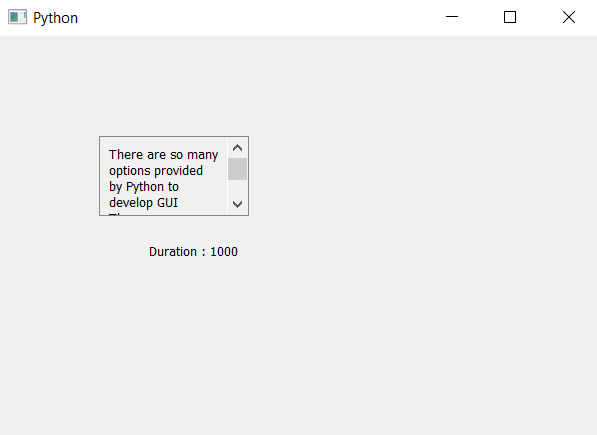# PyQt5 Scrollable Label – Getting tool tip duration

• Last Updated : 03 May, 2020

In this article we will see how we can get the tooltip duration of the scroll label, scrollable label is a label which has a scrolling feature to it.

Steps for implementations –

1. Create a new class which inherits QScrollArea
2. Inside the class create vertical layout
3. Create a label and make it multi-line and add it to the layout
5. Over-ride the setText and text method for label
6. Create object of this class inside the main window class and setting text to it
7. Add tooltip to the object with the help of `setToolTip` method
8. Add tooltip duration to the object with the help of setToolTipDuration method
9. Get tooltip duration of the object with the help of toolTipDuration method
10. Create another label to show the duration

Below is the implementation

 `# importing libraries``from` `PyQt5.QtWidgets ``import` `*` `from` `PyQt5 ``import` `QtCore, QtGui``from` `PyQt5.QtGui ``import` `*` `from` `PyQt5.QtCore ``import` `*` `import` `sys`` ` ` ` `# class for scrollable label``class` `ScrollLabel(QScrollArea):`` ` `    ``# constructor``    ``def` `__init__(``self``, ``*``args, ``*``*``kwargs):``        ``QScrollArea.__init__(``self``, ``*``args, ``*``*``kwargs)`` ` `        ``# making widget resizable``        ``self``.setWidgetResizable(``True``)`` ` `        ``# making qwidget object``        ``content ``=` `QWidget(``self``)``        ``self``.setWidget(content)`` ` `        ``# vertical box layout``        ``lay ``=` `QVBoxLayout(content)`` ` `        ``# creating label``        ``self``.label ``=` `QLabel(content)`` ` `        ``# making label multi-line``        ``self``.label.setWordWrap(``True``)`` ` `        ``# adding label to the layout``        ``lay.addWidget(``self``.label)`` ` `    ``# the setText method``    ``def` `setText(``self``, text):``        ``# setting text to the label``        ``self``.label.setText(text)`` ` `    ``# getting text method``    ``def` `text(``self``):``        ``# getting text of the label``        ``get_text ``=` `self``.label.text()`` ` `        ``# return the text``        ``return` `get_text`` ` ` ` `class` `Window(QMainWindow):`` ` `    ``def` `__init__(``self``):``        ``super``().__init__()`` ` `        ``# setting title``        ``self``.setWindowTitle(``"Python "``)`` ` `        ``# setting geometry``        ``self``.setGeometry(``100``, ``100``, ``600``, ``400``)`` ` `        ``# calling method``        ``self``.UiComponents()`` ` `        ``# showing all the widgets``        ``self``.show()`` ` `    ``# method for widgets``    ``def` `UiComponents(``self``):``        ``# text to show in label``        ``text ``=` `"There are so many options provided by Python to develop GUI "` `\``               ``" There are so many options provided by Python to develop GUI"` `\``               ``" There are so many options provided by Python to develop GUI"`` ` `        ``# creating scroll label``        ``label ``=` `ScrollLabel(``self``)`` ` `        ``# setting text to the label``        ``label.setText(text)`` ` `        ``# setting geometry``        ``label.setGeometry(``100``, ``100``, ``150``, ``80``)`` ` `        ``# setting tool tip``        ``label.setToolTip(``"It is tool tip"``)`` ` `        ``# setting tool tip duration``        ``label.setToolTipDuration(``1000``)`` ` `        ``# getting duration``        ``duration ``=` `label.toolTipDuration()`` ` `        ``# creating another label to show the duration``        ``result ``=` `QLabel(``"Duration : "` `+` `str``(duration), ``self``)`` ` `        ``# setting geometry to the label``        ``result.setGeometry(``150``, ``200``, ``200``, ``30``)`` ` ` ` ` ` `# create pyqt5 app``App ``=` `QApplication(sys.argv)`` ` `# create the instance of our Window``window ``=` `Window()`` ` `# start the app``sys.exit(App.``exec``())`

Output :My Personal Notes arrow_drop_up